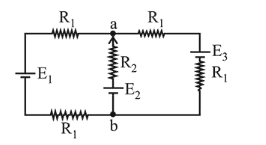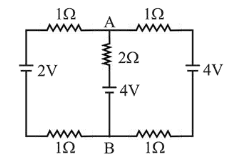# For the circuit shown,Question:

For the circuit shown, with $R_{1}=1.0 \Omega, R_{2}=2.0 \Omega, E_{1}=2 \mathrm{~V}$ and $E_{2}=E_{3}=4 \mathrm{~V}$, the potential difference between the points ' $a$ ' and ' $b$ ' is approximately (in V) :1. (1) $2.7$

2. (2) $2.3$

3. (3) $3.7$

4. (4) $3.3$

Correct Option: , 4

Solution:

(4) Applying parallel combination of batteries Equivalent emf between $\mathrm{A}$ and $\mathrm{B}$ is

$=\frac{\frac{E_{1}}{1+1}+\frac{E_{2}}{2}+\frac{E_{3}}{1+1}}{\frac{1}{1+1}+\frac{1}{2}+\frac{1}{1+1}}$$\frac{\frac{2}{2}+\frac{4}{2}+\frac{4}{2}}{\frac{1}{2}+\frac{1}{2}+\frac{1}{2}}=\frac{5 \times 2}{3}$

$=\frac{10}{3}=3.3$ Volt